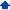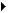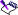Instead of composing formulas, you can use spreadsheet functions. Spreadsheet functions are a set of standard built-in formulas used to simplify complex calculations. Quattro Pro offers more than 500 built-in spreadsheet functions. These spreadsheet functions cover a range of calculations, including database, financial, engineering, and statistical calculations. A common spreadsheet function is @SUM, which adds the cell values you reference. For example, typing @SUM(A1..A4,B1) is equivalent to typing +A1+A2+A3+A4+B1.

For documentation on the available functions for Quattro Pro, see the following sections:

 • Quattro Pro Functions List — provides an alphabetical list of all Quattro Pro functions
 • Quattro Pro Functions Categories — provides a categorized list of all Quattro Pro functions
 • Quattro Pro Functions Equivalents — provides a list of all Quattro Pro functions that differ in name from their Microsoft Excel counterparts

You can enter spreadsheet functions in a cell. As you type a function, the most likely function and its syntax appear at the bottom right of the screen. The function’s arguments will appear on the application bar.

Spreadsheet functions can also be used to create formulas. In a formula, the left parenthesis is red until you type the right parenthesis. Then, they both turn green.

For additional information, see “Rules for entering spreadsheet functions”, “Entering arguments in functions”, and “Working with date functions.”

#### To enter a spreadsheet function in a cell1 Select a cell.
 2 Click InsertInsert function.
 3 Choose a category from the Function category list.
 4 Choose a spreadsheet function from the Function list.
 5 Click Next.
 6 Type the arguments of the function in the relevant boxes; optional arguments appear within angled brackets (<>).• All spreadsheet functions begin with the at sign (@). When typing a spreadsheet function, you can omit the @ if it is not the first item in the formula.
 • Enclose arguments in parentheses.

#### To create a formula by using a spreadsheet function1 Select a cell.
 2 Click InsertInsert function.
 3 Choose a category from the Function category list.
 4 Choose a spreadsheet function from the Function list.
 5 Click Next.
 6 In the Expression box, type the rest of the formula.• The pane on the left shows a breakdown of the formula.
 • To identify a missing parenthesis or bracket, double-click the cell, and move the insertion point through the formula. When you reach an unmatched parenthesis or bracket, the character turns red.